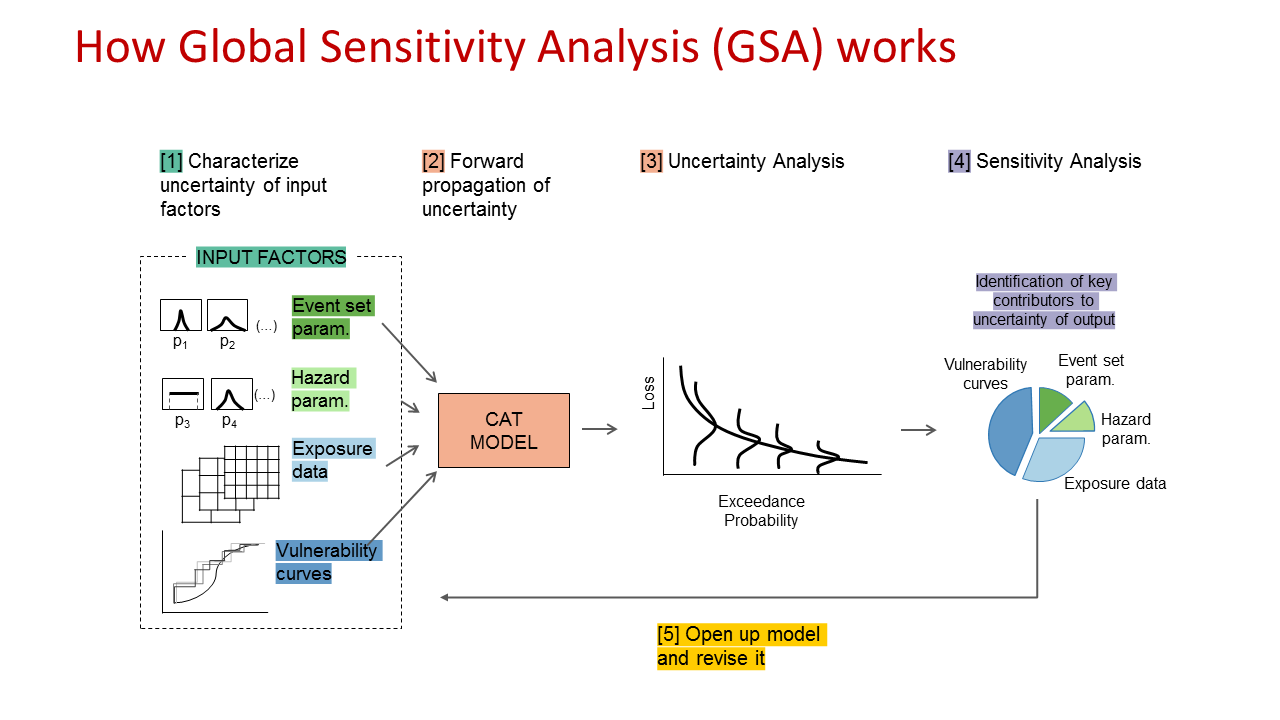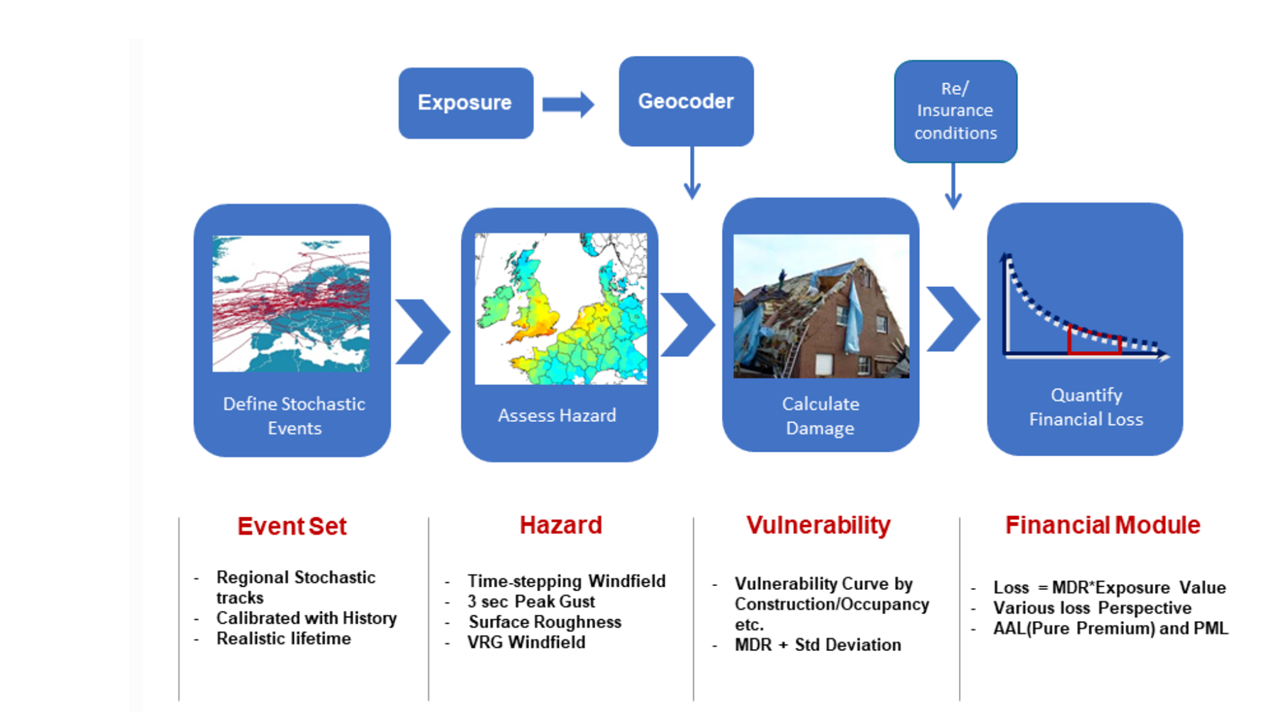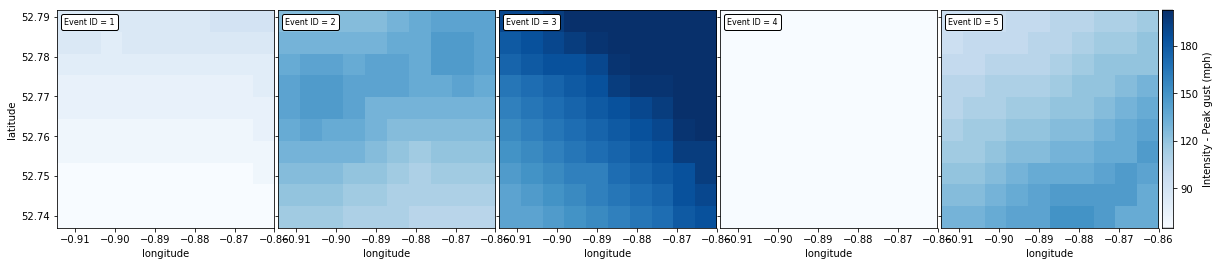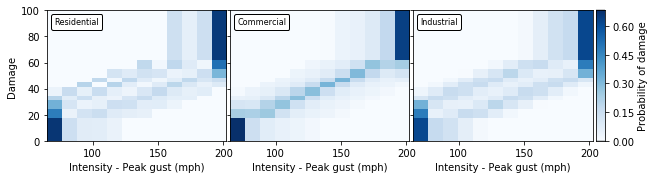In :
from IPython.display import HTML

HTML('''<script>
code_show=true;
function code_toggle() {
if (code_show){
$('div.input').hide(); } else {$('div.input').show();
}
code_show = !code_show
}
</script>

Out:

# Workflow to perform Global Sensitivity Analysis for the PiWind model¶

This document provides:

• a brief introduction to Global Sensitivity Analysis (GSA);
• an introduction to the OASIS LMF PiWind model, which is a toy UK windstorm model (Reference 1);
• a workflow to apply GSA to PiWind using the SAFE (Sensitivity Analysis For Everybody) toolbox (References 2-3).

## What is Global Sensitivity Analysis? and why shall we use it?¶

Global Sensitivity Analysis is a set of mathematical techniques which investigate how uncertainty in the output of a numerical model can be attributed to variations of its input factors.

The benefits of applying GSA are:

1. Better understanding of the model: Evaluate the model behaviour beyond default set-up

2. “Sanity check” of the model: Test whether the model "behaves well" (model validation)

3. Priorities for uncertainty reduction: Identify the important inputs on which to focus computationally-intensive calibration, acquisition of new data, etc.

4. More transparent and robust decisions: Understand the main impacts of input uncertainty on the modelling outcome and thus model-informed decisions

## How Global Sensitivity Analysis works¶

GSA investigates how the uncertainty of the selected model input factors influences the variability of the model output.

An 'input factor' is any element that can be changed before running the model. In general, input factors could be the equations implemented in the model, set-up choices needed for the model execution on a computer, the model's parameters and forcing input data.

In our example of the PiWind model, the input factors subject to GSA will be the wind intensity in the hazard footprint maps; a parameter of the vulnerability curves; and the exposure data in the modelled domain.

An 'output' is any variable that is obtained after the model execution.

In the PiWind model the output is the expected loss due to windstorms.

The key steps of GSA are summarised in the figure below.

Before executing the model, we will sample the inputs from their ranges of variability and then repeatedly run the model so that for each simulation all the inputs vary simultaneously (Input Sampling step). For every output of interest a probability distribution is obtained, after which GSA is performed, to obtain a set of sensitivity indices for each output. The sensitivity indices measure the relative influence of each input factor on the output (References 4,5).

In :
import os
from IPython.display import Image
mydir = "C:/Users/valen/Dropbox/SAFE_work/OasisPiWind"
os.chdir(mydir)
Image(filename="how_GSA_works.png", width=800, height=800)

Out:## What can we learn from GSA?¶

In general, GSA can have three specific purposes:

1. Ranking (or Factor Prioritization) to rank the input factors based on their relative contribution to the output variability. This allows to prioritise resources to reduce uncertainty, so you know on which input factor(s) to focus.

2. Screening (or Factor Fixing) to identify the input factors, if any, which have a negligible influence on the output variability. The input factors which are found to have negligible influence can be fixed to their default values.

3. Mapping to determine the regions of the inputs' variability space which produce output values of interest (e.g. extreme values). For example this can be useful when you want to know which values of your inputs produce an output below or above a threshold of interest.

In :
import pandas as pd
import matplotlib as mpl
import matplotlib.pyplot as plt
import geopandas as gpd
import math
import numpy as np
import json
import seaborn as sns
import folium
from shapely.geometry import Point, Polygon
from descartes import PolygonPatch
from mpl_toolkits.axes_grid1 import AxesGrid
from matplotlib.offsetbox import AnchoredText
import subprocess
import scipy.stats as st
import random
import pickle
import time
import joypy
import warnings

os.chdir(mydir + "/SAFEpython_v0.0.0-alpha/SAFEpython")
# Import SAFE modules:
import RSA_groups as Rg # module to perform RSA with groups
import plot_functions as pf # module to visualize the results
from model_execution import model_execution # module to execute the model
from sampling import AAT_sampling # module to perform the input sampling
from util import aggregate_boot  # function to aggregate the bootstrap results

os.chdir(mydir)


Before applying GSA, let's have an overview of the catastrophe model used here.

## PiWind model structure¶

In :
Image(filename="model_structure.png", width=800, height=800)

Out:PiWind is a wind storm model for a small area of the UK, centred on the town of Melton Mowbray. The data is mocked up to illustrate data formats and functionality, and is in no way meant to be a usable risk model.

Lets plot the area peril cells on a map of the UK. For this model, the area perils are a simple uniform grid in a square.

In :
m = folium.Map(location=[	52.737027, -0.914618], zoom_start=11, tiles='cartodbpositron')
num_cells = area_peril_dictionary.lat.count()
num_cells_per_side = math.sqrt(num_cells)
cell_size_lat = (max(area_peril_dictionary.lat) - min(area_peril_dictionary.lat)) / (num_cells_per_side - 1)
cell_size_lon = (max(area_peril_dictionary.lon) - min(area_peril_dictionary.lon)) / (num_cells_per_side - 1)
for i, row in area_peril_dictionary.iterrows():
geometry = [Polygon([
(row.lon, row.lat),
(row.lon, row.lat + cell_size_lat),
(row.lon + cell_size_lon, row.lat + cell_size_lat),
(row.lon + cell_size_lon, row.lat)])]
crs = {'init': 'epsg:4326'}
d = {'Description': ['All']}
df = pd.DataFrame(data=d)
gdf = gpd.GeoDataFrame(df, crs=crs, geometry=geometry)

m.save("piwind_extent_map.html")

In :
%%HTML
<iframe width="100%" height=350 src="piwind_extent_map.html"></iframe>


## Main components of PiWind:¶

The main components of the PiWind model are:

#### 1. Hazard footprint maps:¶

For each event PiWind generates hazard footprint, which calculates an appropriate hazard metric at each grid point across the entire area effected by the event. For example in the following we will use as hazard metric the maximum 3-second peak gust experienced at every location during the course of the windstorm (stored as “footprint hazard”).

As a matter of example, let's visualise hazard footprints of 5 different events.

In :
footprints_with_hazard = footprints.merge(
intensity_bin_dictionary, how='inner',
left_on='intensity_bin_index', right_on='bin_index').merge(
area_peril_dictionary, how='inner',
left_on='areaperil_id', right_on='areaperil_id')

fig = plt.figure(figsize=(20,10))

grid = AxesGrid(fig, 111,
nrows_ncols=(1, 5),
share_all=True,
label_mode="L",
cbar_location="right",
cbar_mode="single",
)

vmin = min(footprints_with_hazard.interpolation)
vmax = max(footprints_with_hazard.interpolation)
for idx, ax in enumerate(grid):
a = np.zeros([10, 10])
for __, row in footprints_with_hazard[footprints_with_hazard.event_id == idx+1].iterrows():
i, j = row.gridcell.split('-')
a[10-int(i), int(j)-1] = row.interpolation
im = ax.imshow(a, cmap=plt.cm.get_cmap('Blues'), vmin=vmin, vmax=vmax,
extent=(
min(area_peril_dictionary.lon), max(area_peril_dictionary.lon),
min(area_peril_dictionary.lat), max(area_peril_dictionary.lat)))
ax.set_xlabel("longitude")
ax.set_ylabel("latitude")
at = AnchoredText(
"Event ID = {}".format(idx + 1),
prop=dict(size=8),
frameon=True,
loc=2,
)

grid.cax.colorbar(im)
cax = grid.cbar_axes
axis = cax.axis[cax.orientation]
axis.label.set_text("Intensity - Peak gust (mph)")

plt.show()#### 2. Vulnerability curves:¶

Vulnerability curves link the hazard metric (here the 3-second peak gust) to a Mean Damage Ratio (MDR), i.e. the proportion of the total value (e.g. in terms of replacement cost) that would be lost for the asset being analysed. In reality, properties exhibit a high amount of variability in their damage to the same hazard, due to many unknown and un-modellable factors, even when located very close to each other. This is accounted for by defining a probability distribution of losses around the mean damage ratio at each hazard point (this is also known as “secondary uncertainty”).

The PiWind model has seperate vulnerability curves for Residential, Commercial and Industrial occupancies. Let's visualise these curves.

In :
vulnerabilities_with_hazard_and_damage = vulnerabilities.merge(
intensity_bin_dictionary, how='inner',
left_on='intensity_bin_index', right_on='bin_index').merge(
damage_bin_dictionary, how='inner',
suffixes=["_i", "_d"], left_on='damage_bin_index', right_on='bin_index')

fig = plt.figure(figsize=(10,20))

grid = AxesGrid(fig, 111,
nrows_ncols=(1, 3),
share_all=True,
label_mode="L",
cbar_location="right",
cbar_mode="single",
)

vmin = 0.0
vmax = max(vulnerabilities_with_hazard_and_damage.prob)
labels = ["Residential", "Commercial", "Industrial"]
for idx, ax in enumerate(grid):
a = np.zeros((29, 12))
for index, row in vulnerabilities_with_hazard_and_damage[
vulnerabilities_with_hazard_and_damage.vulnerability_id == idx + 1].iterrows():
a[int(row.bin_index_i-1), 11-int(row.bin_index_d-1)] = row.prob

im = ax.imshow(a, cmap=plt.cm.get_cmap('Blues'), vmin=vmin, vmax=vmax,
extent=(
min(intensity_bin_dictionary.interpolation), max(intensity_bin_dictionary.interpolation),
min(damage_bin_dictionary.interpolation) * 100, max(damage_bin_dictionary.interpolation) * 100))

at = AnchoredText(labels[idx],
prop=dict(size=8), frameon=True,
loc=2,
)

ax.set_xlabel("Intensity - Peak gust (mph)")
ax.set_ylabel("Damage")

grid.cax.colorbar(im)
cax = grid.cbar_axes
axis = cax.axis[cax.orientation]
axis.label.set_text("Probability of damage")

plt.show()#### 3. Exposure data:¶

This is model specific logic that maps a set of exposure attributes into the model specific grid and vulnerability type. A unique mapping is made for each location, coverage and peril combination. This also provides informative messages about any exposures that will not be modelled. An exposure may not be modelled if there is insufficiently detailed address information, or if the exposure is not within the geographic scope of the model.

To run the model we need some test exposure data. Lets have a look at an example Location and Account file.

In :
test_locations = pd.read_csv('./tests/data/SourceLocPiWind.csv')

Out:
ACCNTNUM LOCNUM POSTALCODE STATECODE COUNTYCODE LATITUDE LONGITUDE BLDGSCHEME BLDGCLASS OCCSCHEME ... WSCV5DED WSCV6DED WSCV7DED WSCV8DED WSCV9DED WSCV10DED WSSITELIM WSSITEDED WSCOMBINEDLIM WSCOMBINEDDED
0 11111 10002082046 LE13 0HL 1 1 52.766981 -0.895470 RMS 0 R ... 0 0 0 0 0 0 0 0 0 0
1 11111 10002082047 LE13 0HL 1 1 52.766980 -0.895366 RMS 0 R ... 0 0 0 0 0 0 0 0 0 0
2 11111 10002082048 LE13 0HL 1 1 52.766978 -0.895248 RMS 0 R ... 0 0 0 0 0 0 0 0 0 0
3 11111 10002082049 LE13 0HL 1 1 52.766961 -0.895474 RMS 0 R ... 0 0 0 0 0 0 0 0 0 0
4 11111 10002082050 LE13 0HL 1 1 52.766958 -0.895353 RMS 0 R ... 0 0 0 0 0 0 0 0 0 0

5 rows × 50 columns

In :
test_accounts = pd.read_csv('./tests/data/SourceAccPiWind.csv')

Out:
ACCNTNUM POLICYNUM POLICYTYPE UNDCOVAMT PARTOF MINDEDAMT MAXDEDAMT BLANDEDAMT BLANLIMAMT
0 11111 Layer1 2 500000 5000000 0 0 0 0.3
1 11111 Layer2 2 5500000 100000000 0 0 0 0.3

#### 4. Financial module:¶

Calculates losses after taking into account the impact of insurance company policy terms and conditions to provide the net loss that the (re)insurance entity will ultimately be responsible for. The (re)insurance company enters a list of all the policies it has underwritten with information about the location and risk characteristics, such as occupancy type, age, construction material, building height, and replacement cost of the building, as well as policy terms & conditions. The catastrophe model will then run the entire event set across the portfolio, and calculate a loss from every event in the model to every policy.

## Step 1: Define input factors subject to GSA¶

The input factors in this case study are:

• footprint (wind intensity is varied)
• vulnerability (probability of maximum damage is varied)
• exposure (% of building position that are wrong is varied)
In :
# Number of uncertain parameters subject to SA:
M = 3 # (intensity_bin_index (footprint); P(max damage_bin) (vulnerability); SourceLocOEDPiWind10K (exposure);)

# Parameter ranges:
xmin = [0.8, 0.1, 0.1]
xmax = [1.2, 10, 0.5]

# Parameter distributions:
distr_fun =  st.uniform # uniform distribution
# The shape parameters of the uniform distribution are the lower limit and the
# difference between lower and upper limits:
distr_par = [np.nan] * M
for i in range(M):
distr_par[i] = [xmin[i], xmax[i] - xmin[i]]

# Name of parameters (will be used to customize plots):
X_Labels = ['footprint', 'vulnerability','exposure']


## Step 2: Sample inputs space¶

We use random sampling to generate N = 100 combinations of the uncertain input factors. To sample the inputs factors we have defined the distribution of the inputs (uniform) and their range (-20 ÷ 20% for footprint, -20 ÷ 20% for vulnerability and 10 ÷ 50% for exposure)

In :
# sample inputs space
samp_strat = 'lhs' # Latin Hypercube
np.random.seed(1)
N = 100  #  Number of samples
X = AAT_sampling(samp_strat, M, distr_fun, distr_par, N)
np.savetxt("Inputs_100sim_PiWind.csv", X, delimiter=",")


### Examples of varying the input factors¶

In :
Image(filename="perturbed_inputs.png", width=800, height=800)

Out: